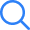# Fluid Mechanics...

TIME LEFT -
• ###### Question 1
2 / -0.33

Oil in a hydraulic cylinder is compressed from an initial volume of 2 m3 to 1.96 m3. If the pressure of oil in the cylinder changes from 40 MPa to 80 MPa during compression, the bulk modulus of elasticity of oil is

• ###### Question 2
2 / -0.33

For a steady two-dimensional flow, the scalar components of the velocity field are Vx = -2x, vy = 2y, vz = 0.The corresponding components of acceleration ax and ay, respectively are;

• ###### Question 3
2 / -0.33

Which of the following conditions is true regarding the validity of Pascal’s Law?

I- It is valid for ideal fluid in motion.

II- It is valid when real fluids with rigid body motion.

• ###### Question 4
2 / -0.33

The velocity in a wind tunnel is being measured using a Pitot-static tube connected to a vertical U-tube manometer. The density of air is 1.2 kg/m3 and the deflection of the manometer is 24 mm. The manometer fluid is water. The velocity measured by the Pitot-static tube is

• ###### Question 5
2 / -0.33

A centrifugal pump lifts water through a height h and delivers it at a velocity vd. The loss of head through piping is hf. The gross lift is

• ###### Question 6
2 / -0.33

Select the correct option for the velocity profile

$$\frac{u}{V} = \frac{5}{2}\left( {\frac{y}{\delta }} \right) - \frac{1}{2}{\left( {\frac{y}{\delta }} \right)^2} + \frac{2}{3}{\left( {\frac{y}{\delta }} \right)^3}$$

• ###### Question 7
2 / -0.33

The velocity field for a 2 D, steady and incompressible flow is represented as V = (5x) i – (5y) j. What would be the equation of stream line passing through point (1, 1).

• ###### Question 8
2 / -0.33

Oil (kinematic viscosity, νoil = 1.0 × 10-5 m2/s) flows through a pipe of 0.5 m diameter with a velocity of 10 m/s. Water (kinematic viscosity, vw = 0.89 × 10-6 m2/s) is flowing through a model pipe of diameter 20 mm. For satisfying the dynamic similarity, the velocity of water (in m/s) is _______.

• ###### Question 9
2 / -0.33

The x and y - components of the velocity field for a two dimensional fluid flow is given by u = 3y – y2 and v = 3x - x2. What would be the rate of shear deformation and angular velocity of fluid flow in z direction respectively at point (2, 3)?

• ###### Question 10
2 / -0.33

A smooth flat plate of length 5 m and width 2 m is moving with a velocity of 4 m/s in stationary air of density 1.25 kg/m3 and kinematic viscosity 1.5 × 10-5 m2/s. The total drag force (N) on one side of the plate assuming that the boundary layer is turbulent from the very beginning is _________.

(Correct up to 2 decimals)

Assume for turbulent flow over a flat plate, local drag coefficient is:

$${{C}_{{{D}{,}}x}}=\frac{0.059}{Re_{x}^{1/5}}$$

Submit Test##### Question Analysis

• 1
• 2
• 3
• 4
• 5
• 6
• 7
• 8
• 9
• 10
Submit Test×
Open Now